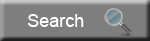Online Hydro Steering Calculator By Bill "BillaVista" Ansell Java Programming: Chad Lloyd Photography: Bill Ansell Copyright 2003 - Bill AnsellYellow values are entered by user.

Green values are calculated

Simply enter the required values (the one's with the yellow labels) and the calculator will automatically update.

 Step 1 - Calculate Approximate Kingpin Tourque (in/lbs) Weight on Steered Axle (lbs) Coefficient of Friction Nominal Tire Width (in) Kingpin Offset at Ground Intersection (in) DriveFactor (2 = steered wheels driven, 3 = locked) Kingpin Tourque (in/lbs) Step 2 - Calculate Approximate cylinder force Minimum Radius Arm (in) Cylinder Force (lbs) Step 3 - Determine pressure rating of system Pressure rating of pump (psi) Step 4 - Calculate required Cylinder Area (sq. in) Cylinder Area (sq. in) Step 5 - Determine type of cylinder used Style (1 = single balanced) (2 = single unbalanced) (3=cross connected unbalanced) Step 6 - Determine diameter of shaft (rod) of cylinder(s) Shaft diameter (in) Step 7 - Determine required cylinder stroke (in) Cylinder Stroke (in) Step 8 - Calculate approximate required cylinder bore (in) Cylinder Bore (in) Step 9 - Calculate swept Volume of Cylinder(s) (cu. in) Bore diameter (in) Swept Volume (Cu. in.) Step 10 - Calculate required steering unit Number of turns lock to lock Valve displacement required (cu. in./rev) Step 11 - Determine displacement of nearest steering unit Valve displacement of actual unit (cu. in./rev) Step 12 - Calculate turns from lock to lock Turns lock to lock (One Way) Turns lock to lock (Other way) Step 13 - Calculate minimum pump flow (gpm) Fastest Steering speed required (rev/sec) Minimum Pump Flow Required (gpm)Total:Get a GoStats hit counter BillaVista.comGet a GoStats hit counter